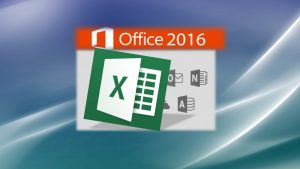###Excel for the Workplace – Advance in your Career

A self-paced course on using Microsoft Excel in the workplace.

### What you will learn:

• Master Microsoft Excel from Beginner level to Intermediate level.
• Learn the most commonly used Excel functions in today’s business environment.
• Master unique tips and concepts that you won’t find in other courses.
• Build a solid understanding of the basics of Microsoft Excel to increase your proficiency at work.
• Create dynamic charts and data analysis using IF, VLOOKUP, and PivotTables.
• Access  to a Professional Trainer with 15+ years of Excel and IT experience.

### Requirements

• Install Microsoft Office: Excel 2016, 2013 or 2010. The course is designed using Excel 2016.
• No previous knowledge is required!
• Great for students or employees who want to learn at their own pace

### Certificate

A Verifiable Certificate of Completion is presented to all students who request it upon completion of this course.

### Application

This course is suitable for individuals without any previous experience in Excel. We will start from the very basics and will gradually move on to some of the more advanced features, such as lookup functions, functions with conditions, pivot tables, custom formatting, Excel 2016 charts, and other tools that are often used the business environment.

This course is perfect employee training for small and midsize businesses and for individuals such as  students, entry-level finance, Business and Marketing professionals who would like to grow faster than their peers.

• Executive Assistant
• Office Clerk
• Auditor
• Accountant
• Financial Analyst
• Credit Counselor
• Sales Representative
• Sales Manager
• Financial Manager
• Investment Banker
• Real Estate Agent
• Market Research Analyst
• Entrepreneur
• Someone who is involved with a business and would like to be successful
• Students

Please don’t forget that the course comes with an unconditional money-back-in-full guarantee. And why not give such a guarantee, when we are convinced that it will provide a ton of value for you?

### Unit Sections and Objectives

Lesson 1: Getting Started with Excel – General Concepts

• Getting started with Excel
• General interface
• The Office Ribbon
• General concepts on using Excel and Spreadsheets

Lesson 2: Calculating the SUM, AVERAGE etc. in Excel

• How to use Excel basic features
• How to enter a formula in Excel
• How to calculate the sum of a series of cells
• How to calculate the average of a series of cells
• How to calculate the Max of a series of cells
• How to calculate the Min of a series of cells
• How to calculate the count function in a series of cells
• How to use the Autofill feature in Excel

Lesson 3: Basic Formulas: Multiplying, Division and Subtraction in Excel

• Basic arithmetic functions in Excel
• Subtraction
• Multiplying
• Dividing using formulas

Lesson 4: Absolute, Relative and Mixed References

• How and when to use different types of references in formulas in Excel.
• Difference between absolute and relative references
• Mixed references

Lesson 5: Using Conditional Formatting and Data Analysis in Excel

• How to apply formatting styles to the data in a spreadsheet.
• How to apply conditional formatting to selected data
• How to use Data Analysis in Excel

Lesson 6: Data Sorting and Filtering in Excel

• How to sort data in Excel
• How to filter data based on various criteria
• How to customize the filters
• How to clear the filters
• How to use sorting and filtering by right clicking

Lesson 7: Calculating Percentages in Excel

• How to calculate percentages using a formula in Excel

Lesson 8: Creating and Customizing Charts in Excel

• How to create various types of charts in Excel
• How to customize the charts
• Which types of charts to use for certain types of data

Lesson 9: Cross-Sheet Calculations in Excel

• How to use formulas to perform cross-sheet calculations in Excel
• How to enter the value from a formula in a previous sheet in a new worksheet.

Lesson 10: Using the IF Function in Excel

• How to use the IF Statement or function in a variety of ways in Excel 2013 via a formula

Lesson 11: Using Named References in Excel

• How to define Named References in Excel.
• How to apply Named References and how to use this important feature in Excel.

Lesson 12: Using Data Validation and Dropdown Lists in Excel

• How and why use data validation in Excel
• How to define data validation rules and criteria.
• How to apply data validation lists and drop-down lists

Lesson 13: Using Pivot Tables in Excel

• How to setup the data to use it with Pivot Tables.
• How to create pivot tables in Excel
• How to customize the pivot tables using various criteria.

Lesson 14: Setting the Print Area in Excel

• Setting and clearing the print area.

Lesson 15: Linking Data from Excel with Word for Reports

• How to link data from Excel into Word for reports etc. using Paste Special.
• Update the data in your Excel spreadsheet and have that data updated automatically in Word.

Lesson 16: Importing and Exporting Data in .csv Files in Excel to Transfer Data Between Systems

• Understanding .csv files and their usage.
• How to import .csv files in Excel
• How to export an Excel file to .csv format.

Lesson 17: Sharing and Saving Data in PDF Format

• How to share data files in Excel.
• How to export data in PDF format directly from Excel

Lesson 18: Creating a Mail Merge using Excel

• How to perform a mail merge using an Excel Data File

Lesson 19: Using VLOOKUP in Excel with 5 examples

### Questions?

Not readable? Change text.